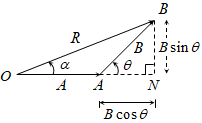# the resultant of two forces 3P and 2P is R. If the first force is doubled then the resultant is also doubled. then the angle between the two forces us. plz xplain with the soln..

9 years ago

Dear Nandini,

In 1st case, R2=(3P)2+(2P)2+2*3*2*P2*cosΘ = 9P2+4P2+12P2cosΘ = 13P2+12P2cosΘ ----->(1)

In 2nd case, (2R)2=(6P)2+(2P)2+2*6*2*P2*cosΘ ; 4R2=36P2+4P2+24P2cosΘ = 40P2+24P2cosΘ ------->(2)

Multiply (1) by 4; 4R2=52P2+48P2cosΘ ------>(3)

(3)-(2) gives, 0=12P2+24P2cosΘ

Therefore, cosΘ = (-1/2) => Θ = cos-1(-1/2) = 120°

9 years ago

case 1, R2=(3P)2+(2P)2+2*3*2*P2*cosΘ = 9P2+4P2+12P2cosΘ = 13P2+12P2cosΘ ----->(1)

case 2, (2R)2=(6P)2+(2P)2+2*6*2*P2*cosΘ ; 4R2=36P2+4P2+24P2cosΘ = 40P2+24P2cosΘ ------->(2)

Multiply (1) by 4; 4R2=52P2+48P2cosΘ ------>(3)

(3)-(2) gives, 0=12P2+24P2cosΘ

Therefore, cosΘ = (-1/2) => Θ = cos-1(-1/2) = 120°

9 years ago

r2=9p2+4p2+2.6p2cosθ....................1

4r2=(6p)2+4p2+2.12cosθ..................2

dividing 2 from 1

4=(36+4+24cosθ)/(9+4+12cosθ)

9+4+12cosθ=10+6cosθ

6cosθ=-3

cosθ=-0.5

θ=120degreeKushagra Madhukar
2 years ago
Dear student

Let us assume, 3P = A; and, 2P = B, which makes an angle θ with each.Now, according to law of vector addition,
R2 = A2 + B2 + 2ABcosθ
or, R2 = (3P)2 + (2P)2 + 2(3P)(2P)cosθ = 13P2 + 12P2cosθ          --- (1)

as given, when A is replaced by 2A (= 6P), resultant vector also gets doubled.
Hence, new resultant R’ = 2R
Therefore,
(2R)2 = (6P)2 + (2P)2 + 2(6P)(2P)cosθ
or, 4R2 = 40P2 + 24P2cosθ

substituting the value of R2 from eqn. (1), we have,
4[ 13P2 + 12P2cosθ ] = 40P2 + 24P2cosθ
or, 24P2cosθ = – 12P2
or, cosθ = -1/2
or, θ = cos-1(-1/2) = 120o
Hence, the angle between the vectors is, θ = 120o

Hope it helps.
Regards,
Kushagra